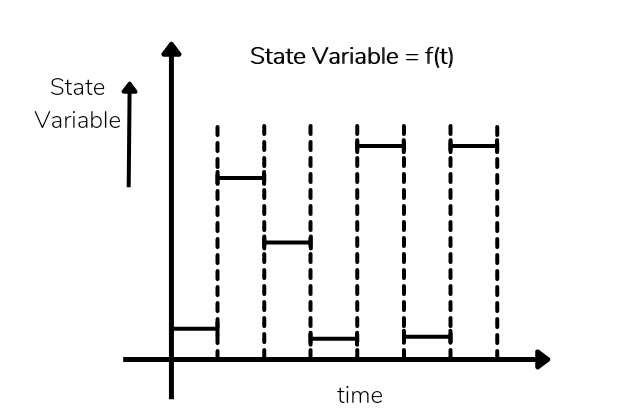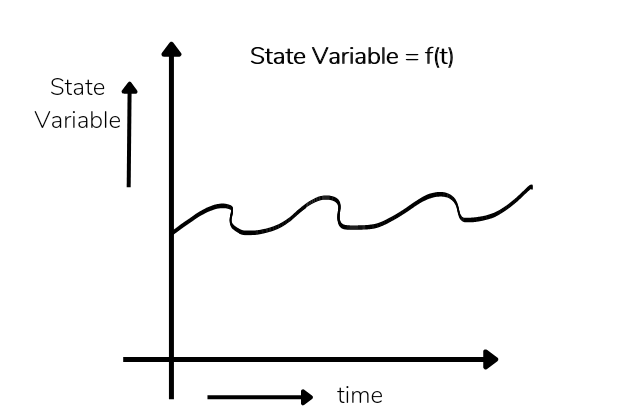# Discrete and Continuous System in Simulation.

### Discrete System Simulation

In a system when state variables change only at a countable number of points in time time is called discrete model system.

Discrete system, state variable change at discrete point in time.The point in time were state variable change is called event occur or change in state.

Discrete model having finite number of states.

In discreet system time change in incremental steps.

District model is used to solve numerical method like computational procedures

Example queue length at a cash cash machine.

### Continuous System Simulation

In a system when state variables change in a continuous way and not abruptly from one state to another that is called continuous system.

In continuous system, state variables continuously as a function of time.#### ✓Continuous Model Behaviour

Continuous model having infinite number of steps. it means state variables change infinite time in continuous model.

In continuous model time change continuously.

Continuous model is used to solve ore define analytical method like deductive mathematical reasoning.

Ex. flow of air around a car.